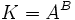# Normality is not transitive for any pair of nontrivial quotient groups

## Statement

Suppose$A$ and$B$ are (possibly equal) nontrivial groups. Then, there exist groups$H \le K \le G$ such that all the following conditions are satisfied:

•$H$ is a Normal subgroup (?) of$K$ and the quotient group$K/H$ is isomorphic to$A$.
•$K$ is a normal subgroup of$G$ and the quotient group is isomorphic to$B$.
•$H$ is not a normal subgroup of$G$.

## Proof

The construction is as follows. Let$G$ be the wreath product of$A$ and$B$ for the regular group action of$B$. Let$K$ be the subgroup$A^B$, i.e., the normal subgroup that forms the base of the semidirect product, and let$H$ be the subgroup of$K$ where a particular coordinate is the identity element. (If we are thinking of$A^B$ as functions from$B$ to$A$, then$H$ can be taken as the subgroup comprising those functions that send the identity element of$B$ to the identity element of$A$ -- here, the particular coordinate becomes the coordinate corresponding to the identity element of$B$).

Thus,$K$ is isomorphic to$H \times A$. Then:

•$H$ is normal in$K$ and$K/H$ is isomorphic to$A$: PLACEHOLDER FOR INFORMATION TO BE FILLED IN: [SHOW MORE]
•$K$ is normal in$G$ and$G/K$ is isomorphic to$B$: PLACEHOLDER FOR INFORMATION TO BE FILLED IN: [SHOW MORE]
•$H$ is not normal in$G$: The action of$B$ on the coordinates in$K = A^B$ is transitive on the coordinates, so$H$ is not preserved under this action.